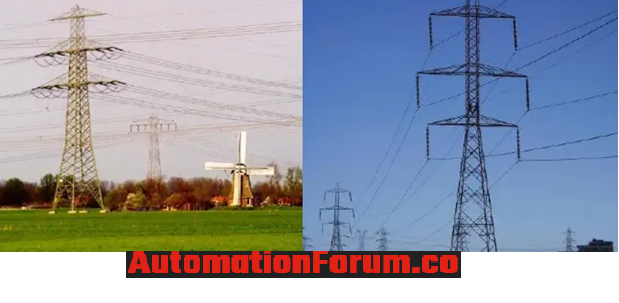# How to solve the voltage drop problems over the long-distance cable? How to calculate the voltage drop?In order to do the distribution of electric power from generating stations to the consumer long-distance power transmission is needed and for this purpose we use transformers. The power is transmitted from the generating stations to the consumer by using transmission lines and we select conductors that have less resistance and the power transmission is done in high voltages. Basically, the voltage drop can be described as the reduction of the voltage in the supplied energy due to the movement of the electric current through the wires or any other components.

### What could happen if we use long cables for power distribution?

In order to do the long-distance transmission, we must increase the length of the cable and because of this the cable resistance also increases. Because of this the load due to the resistance also increases and this load will create a voltage drop in the line and a percentage of the voltage will be lost in the line. So when the resistance increases then the load increases.

### Why there is less voltage drop in higher size cables?

In a higher size cable, the cross-sectional area of the conductor is high when compared to a lower size cable and because of this the resistivity of the higher size cable is less and due to this the load across the cable will decrease and the voltage drop will decrease too. So higher size cables have less resistance than the lower size cables.

### How to calculate the Voltage drop?

Voltage drop calculation

Voltage drop = (milli volt × ampere load × length of the cable) ÷ 1000

Voltage drop for single phase = (VD × 100) ÷ 240

Voltage drop for three phase = (VD × 100) ÷ 415

The millivolt is the millivolt per ampere per meter is the value that would be given by the cable manufacturer, so check the datasheet to get the value. Load ampere is the required current and the cable length is the length of the distribution line.

### What are the problems that can be caused because of the voltage drop?

As per the electrical standard, the voltage drop must be less than 3% so if we have a voltage drop of 5% then it is a problem. As per the above details and formulas, we can calculate the voltage drop in single-phase and three-phase. So if the calculated voltage drop is more than 3% it could badly affect the equipment, at first there won’t be any problem the device would work properly but after a period of time, it could be damaged so voltage drop is an important factor that must be considered while power distribution.

### What are the advantages of high voltage power transmission?

The voltage drop would be less for a higher voltage circuit for the given power. The major advantage of high voltage transmission is that it can carry more power than the given ampacity. Line loss would be less for high voltage transmission. Due to the less voltage drop and high capacity, high voltage power transmission can cover a wider area. Less substation is required because of the longer reach.

Scroll to Top